Next: Equal Subcycled to Non-Subcycled Up: 2-D ANALYSIS AND RESULTS Previous: 2-D ANALYSIS AND RESULTS   Contents

Multi-Time Step Subcycling Results

In order to verify the 2-D nodal subcycling algorithm describerd in Chapter 2, we test it on a homogeneously discretized mode I crack problem presented in Figure 4.1. The middle region contains cohesive elements which define it as the critical or subcycled region, since the overall domain time step must be reduced due to the cohesive elements. The mode I loading is due to an applied velocity of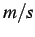on the top and bottom of the domain.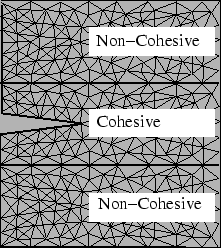The entire domain is composed of PMMA material with a Young's Modulus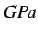, Poisson's ratio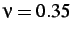, and density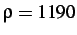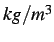. The cohesive elements have a maximum stress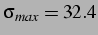, initial strength parameter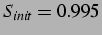and a normal and tangential critical separations of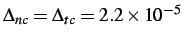. The time step is reduced by thirty to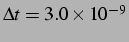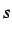, and the simulation is run for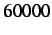time steps, or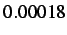, on a Pentium III, 600 MHz, 750Mb RAM processor, running Mandrake Linux 7.2.

In our primarily analysis, we wish to see the effect that the ratio of non-subcycled to subcycled nodes - also known as the region ratio - has on the timing and accuracy of the solutions. We test the algorithm for region ratios of ,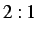and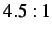. For each of these cases we use subcycling parameters of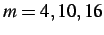and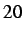in the non-critical regions of the domain. Optimally, we should be able to use a maximum ofsince we reduce the original time step by this amount. Previous results have shown that the optimal parameter cannot be achieved due to the severe oscillations that occur, as a result we maximize our parameter at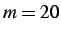.

Based on our results in 1-D, for low low region ratios as well as low subcycling parameters, the cost of the algorithm implementation can potentially offset the savings gained through subcycling. In increasing both of these ratios we will show that the computational savings is increased although the accuracy of the solutions decreases with the higher subcycling parameters.

SubsectionsNext: Equal Subcycled to Non-Subcycled Up: 2-D ANALYSIS AND RESULTS Previous: 2-D ANALYSIS AND RESULTS   Contents
Mariusz Zaczek 2002-10-13